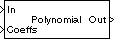# Polynomial Evaluation

Evaluate polynomial expression

## Library

Math Functions / Polynomial Functions

`dsppolyfun`

•## Description

The Polynomial Evaluation block applies a polynomial function to the real or complex input at the `In` port.

```y = polyval(u) % Equivalent MATLAB code ```

The Polynomial Evaluation block performs these types of operation more efficiently than the equivalent construction using Simulink® Sum (Simulink) and Math Function (Simulink) blocks.

When you select the Use constant coefficients check box, you specify the polynomial expression in the Constant coefficients parameter. When you do not select Use constant coefficients, a variable polynomial expression is specified by the input to the `Coeffs` port. In both cases, the polynomial is specified as a vector of real or complex coefficients in order of descending exponents.

The table below shows some examples of the block's operation for various coefficient vectors.

Coefficient VectorEquivalent Polynomial Expression
`[1 2 3 4 5]`

$y={u}^{4}+2{u}^{3}+3{u}^{2}+4u+5$

`[1 0 3 0 5]`

$y={u}^{4}+3{u}^{2}+5$

`[1 2+i 3 4-3i 5i]`

$y={u}^{4}+\left(2+i\right){u}^{3}+3{u}^{2}+\left(4-3i\right)u+5i$

Each element of a vector or matrix input to the `In` port is processed independently, and the output size is the same as the input.

## Parameters

Use constant coefficients

Select to enable the Constant coefficients parameter and disable the `Coeffs` input port.

Constant coefficients

Specify the vector of polynomial coefficients to apply to the input, in order of descending exponents. This parameter is enabled when you select the Use constant coefficients check box.

## Supported Data Types

• Double-precision floating point

• Single-precision floating point

 Least Squares Polynomial Fit DSP System Toolbox Math Function (Simulink) Simulink Sum (Simulink) Simulink `polyval` MATLAB

## Extended Capabilities

### C/C++ Code GenerationGenerate C and C++ code using Simulink® Coder™.

Introduced before R2006a

Watch now